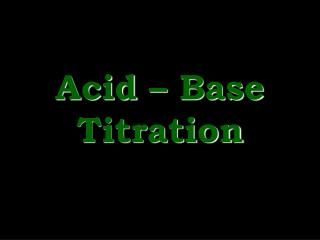DownloadDownload PresentationAcid – Base Titration

# Acid – Base Titration

Télécharger la présentation## Acid – Base Titration

- - - - - - - - - - - - - - - - - - - - - - - - - - - E N D - - - - - - - - - - - - - - - - - - - - - - - - - - -
##### Presentation Transcript

1. Acid – Base Titration

2. Prelab question MA x #H x VA = MB x #OH x VB MA = 6 M, #H = 2, VA = ? MB = 0.5 M, #OH = 1, VB = 0.5 L (6 M)(2)(VA) = (0.5 M)(1)(0.5 L) VA = (0.5 M)(1)(0.5 L) / (6 M)(2) VA = 0.25 mol / 12 M = 0.0208 L = 20.8 mL

3. Typical results from experiment

4. Calculations (0 – 25) 0.025 0.035 0.040 0.045 0.048 0.049 0.050 0 .0020 .0030 .0040 .0046 .0048 .0050 .0050 .0030 .0020 .0010 .0004 .0002  0  0  0 0.200 0.086 0.050 0.022 0.008 0.004 0.70 1.067 1.301 1.653 2.079 2.389 7  0 13.3 12.9 12.7 12.3 11.9 11.6 7

5. Calculations (25 – 50) 0.050 0.051 0.052 0.055 0.060 0.065 0.075 .0050 .0052 .0054 .0060 .0070 .0080 .0100  0 .0050  0 .0002 .0004 .0010 .0020 .0030  0 7  0 .0039 .0077 .0182 .0333 .0462 .0667 7 2.4 2.1 1.7 1.5 1.3 1.2

6. Predicted results

7. 3. Titration: the combination of two solutions in the presence of an indicator; often used to determine the unknown concentration of one of the solutions. Endpoint: the point when the indicator colour changes (usually the end of the titration) Equivalence point: The point when the number of equivalents mixed together are the same (in acid-base titrations equivalents refer to the number of moles of H+ and OH–) • At 25 mL HCl added the pH changes very rapidly, thus a small error in this region would be the easiest point to get an incorrect value.

8. 5 A) 14 14 7 7 0 0 0 0 25 25 50 50 Fewer moles of H2SO4 would be required to neutralize 25 mL NaOH, thus the curve would shift left 5 B) Because we start with pure HCl, then add NaOH the pH starts low then goes high, thus the curve flips

9. 5 C) 14 14 7 7 0 0 0 0 25 25 50 50 A weak base would lower the “base” part of the curve (it’s weak so it has a lower pH), thus yielding a lower (acidic) equivalence point 5 D) A weak acid would raise the “acid” part of the curve (it’s weak so it has a higher pH), thus yielding a higher (basic) equivalence point

10. For more lessons, visit www.chalkbored.com • Refer to table 15.3 (pg. 606) • Bromothymol (6.0 – 7.6) is a better choice than phenolphthalein (8.2 – 10.0) because it’s colour change occurs at a pH that is closer to the equivalence point (7.0) • Phenolphthalein is often used because it is more colourful (thus easier to see) • Any indicator that is close to the expected equivalence point (in this case at a pH of 7) is an acceptable choice. • e.g. Bromocresol purple (5.2 – 6.8), Litmus (4.7 – 8.3), Cresol red (7.0 – 8.8), etc. • Note: A narrow pH range is also preferable.